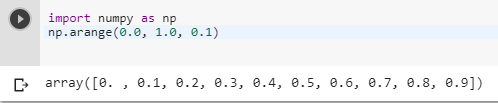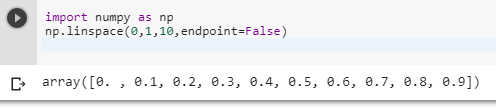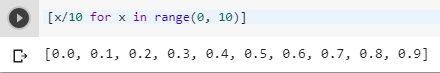2 views
in Python

Is there a way to step between 0 and 1 by 0.1?

I thought I could do it like the following, but it failed:

for i in range(0, 1, 0.1):

print i

Instead, it says that the step argument cannot be zero, which I did not expect.

by (106k points)

We have many ways to get the decimal point value but what you are doing is not supported in Python:-

To use a floating-point step value, you can use the arange() function for that you need to import numpy library.

Below is the code that show how to do it:-

import numpy as np

np.arange(0.0, 1.0, 0.1)But the best thing you can do to get decimal values is to use the linspace function from the NumPy library (which isn't part of the standard library but is relatively easy to obtain). linspace takes a number of points to return and also lets you specify whether or not to include the right endpoint.

Below is the code that illustrates how to do it:-

import numpy as np

np.linspace(0,1,10,endpoint=False)Another alternative way is to use list comprehension. What you were doing is using Python's range() function which can only do integers, not floating point. In your specific case, you can use a list comprehension instead.

[x/10 for x in range(0, 10)]